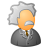# QlikView App Dev

Discussion Board for collaboration related to QlikView App Development.

Announcements
Join this live chat April 6, 10AM EST - QlikView to Qlik Sense REGISTER
cancel
Showing results for
Search instead for
Did you mean:Partner

## Cumulative Month Issue

I am using a set analysis expression and my data is a Month to Date Data. I want to calculate the YTD  up to the current month my date format is 'YYYYMM'

I have tried the following :

sum({\$<CalendarYear = {\$(=Max(CalendarYear)-1)},CalendarMonthName={"<=\$(vMonthToday()"}>} Sales)

where vMonth is my variable defined as Month(Today()).

Can anyone please help.

5 RepliesMVP

If this is working for you

sum({\$<CalendarYear = {\$(=Max(CalendarYear)-1)},CalendarMonthName={"<=\$(vMonthToday()"}>} Sales)

Then, YTD may be this?

Sum({<Year=, Month=, Quarter=, Week=, CalendarYear={">=\$(=Num(YearStart(Max(CalendarYear))))<=\$(vMonthToday)"}>} Sales)

But, If you ask please share YTD formula for that expression i would write like below

Sum({<Year=, Month=, Quarter=, Week=, CalendarYear={">=\$(=Num(YearStart(Max(DateField))))<=\$(=Num(Max(DateField)))"}>} Sales)

Before develop something, think If placed (The Right information | To the right people | At the Right time | In the Right place | With the Right context)Partner
Author

NO my original expression is not working that is why I posted itSpecialist

may be this for current YTD

sum({\$<Date={"<=\$(=date(today(),'YYYYMM'))>=\$(=date(yearStart(today()),'YYYYMM'))"}>} Sales)MVP

May be try this?

sum({\$<CalendarYear = {">= \$(=Date(Max(CalendarYear),'YYYYMM')-1) <=\$(=Date(Month(Today()),'YYYYMM'))"}>} Sales)

Before develop something, think If placed (The Right information | To the right people | At the Right time | In the Right place | With the Right context)Partner
Author

Sorry doesn't work

=sum({\$<CalendarYear = {">= \$(=Date(Max(CalendarYear),'YYYYMM')-1) <=\$(=Date(Month(Today()),'YYYYMM'))"}>}  Sales)

We are in June right now, So I would like to compare June /2017 and June 2016,